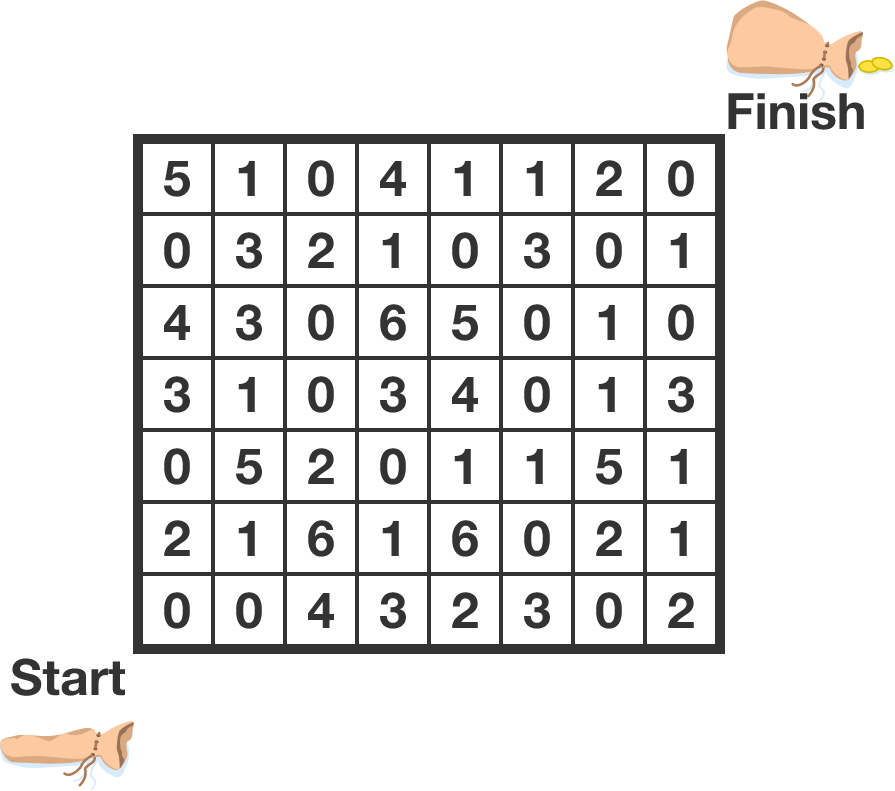# Maximize the GoldIn the grid above, the number of gold coins in each cell is given. Adam needs to start in the bottom-left square and move to the top-right square, moving only up and to the right.

What is the maximum number of gold coins he can collect?

This can be solved using a recursive approach (dynamic programming).

• I've set up the grid (coins), where the bottom-left cell is (0,0) and the top-right is (7,6); i.e., coins[(1,2)] = 5.
• I've also computed max_coins, the maximum number of coins Adam can collect along the way to a given cell, for the first column and row.
• You need to fill in the logic in line 19 - the recursive step for max_coins for the rest of the cells - and run the code!

Hint: What are the possible states just before you reach a given cell?

from brilliant import goldgrid
coins = goldgrid.grid()
height, width = goldgrid.height(coins), goldgrid.width(coins)

#Initializing the dictionary for the maximum coins up to a certain cell
max_coins = {(0,0) : coins[(0,0)]}

#Computing the max_coins values for the first column and row
#The maximum coins is the maximum for the cell prior to it, plus the coins in that cell
for row in range(1,height):
max_coins[(0,row)] = max_coins[(0,row-1)] + coins[(0,row)]

for column in range(1,width):
max_coins[(column,0)] = max_coins[(column-1,0)] + coins[(column,0)]

#Using recursion / dynamic programming to find the remaining maximums
for row in range(1,height):
for column in range(1,width):
max_coins[(column,row)] = #FILL ME IN

#Print the maximum value for the top right cell
print(max_coins[(width-1,height-1)])
Python 3
You need to be connected to run code

Bonuses: How can you initialize max_coins differently to avoid the separate cases for the first column and row, and just have a single recursive loop? Also, can you modify the code to return the actual path that gives the maximum gold?

×